• 我们先来看图，看看这个方法的操作过程，等一下，我找找我的大学的线性代数课本，找到啦！（哈哈，虽然读研了，因为我是...1.首先我们看看这个正交化过程，因为a1,a2...an为一向量（大佬们请原谅我用a字母代替阿...
我们先来看图，看看这个方法的操作过程，等一下，我找找我的大学的线性代数课本，找到啦！（哈哈，虽然读研了，因为我是菜鸟，所以还是随时带着）如下图所示：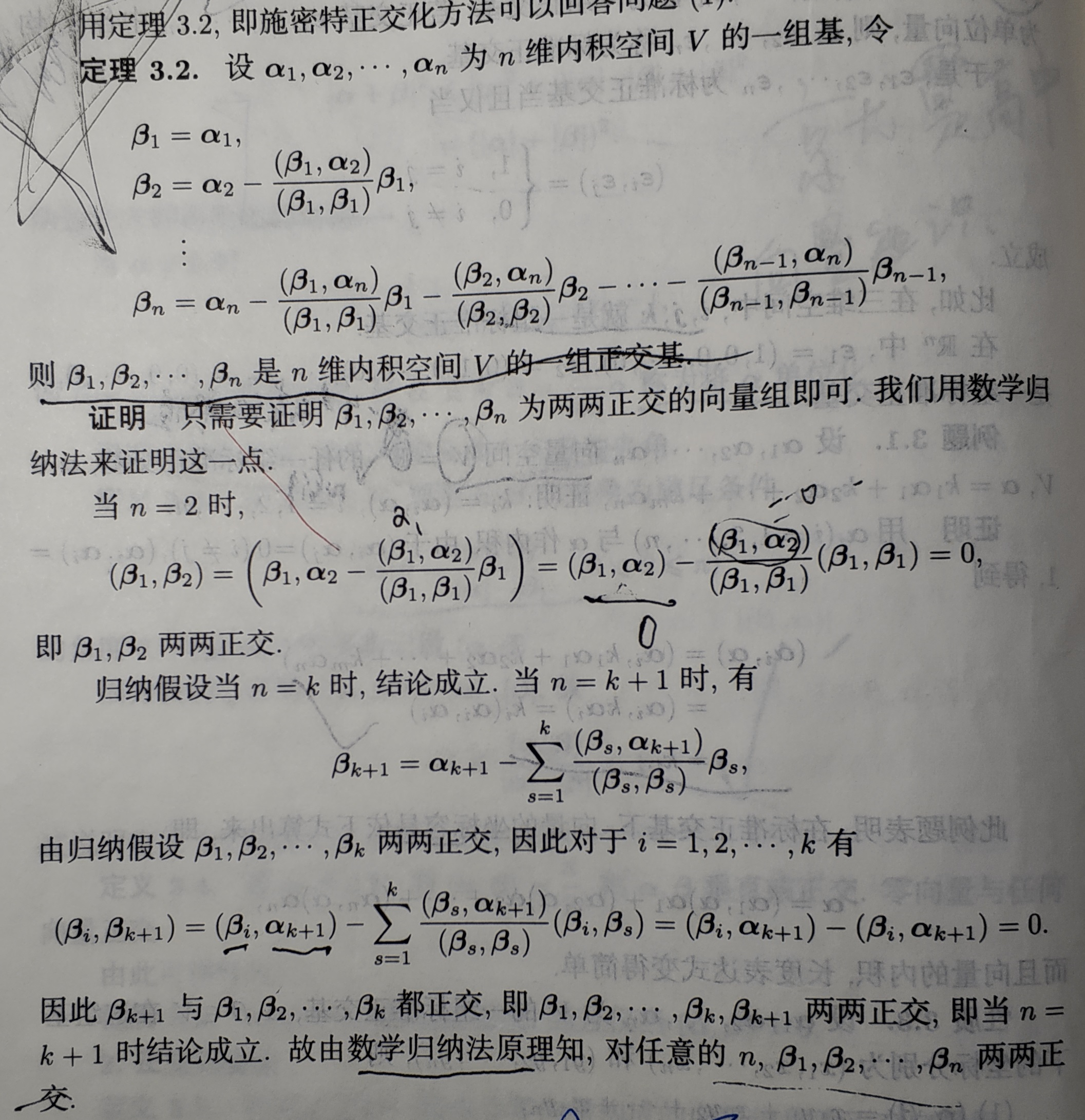大部分人在考研时候都是直接背下来这个正交化过程对吧，或者也根本没有搞懂为啥这样操作就能够得到正交化的基，现在就结合我的理解来分析一下这个原理吧
1.首先我们看看这个正交化过程，因为a1,a2...an为一组基向量（大佬们请原谅我用a字母代替阿尔法希腊字母，实在是太难输入了，嘻嘻！），只是满足k1a1+k2a2+k3a3+...knan = 0时候，一定有系数k1=k2=...=kn=0，但是如果，a1,a2...an没有任意两者内积为零，也就是他们不是正交的，这就说明了一个问题，a1,a2...an这些基向量之间有冗余（如果是正交基的话，应该是不存在这个问题的），意思就是他们之间的一些分量可能重叠了，还不理解?（我以前学习的时候听到这些也是一脸蒙逼）哈哈，没关系，那我就来举个例子吧，我们看下面的例子：
情况一：假如：
a1 = (1,0) , a2 = (0,1)
我们先来测试他是不是一组基（这两个向量也太简单了，劳资一眼就能看出来是吧，哈哈，我们假装不知道，人生不易，全靠演技！），上面我说了，是不是一组基就看他是不是线性无关，即：
k1a1+k2a2 = 0,看看是否k1=k2=0,如果k1=k2=0,那么a1,a2线性无关，否则就是线性相关了
我们带入进去看看
k1(1,0)+k2(0,1) = 0，很明显了，k1=k2=0，所以a1,a2线性无关，而且a1*a2 = 0，（这里面*都表示向量内积）所以a1垂直a2，即a1,a2正交，那么说明a1,a2是一组正交基，
又因为a1,a2的模长（就是向量所确定的点到原点的距离）为1的，
即|a1|=|a2|=1，所以a1,a2是一组标准正交基（有的书可能叫做规范正交基）
情况二：现在我再假如：
a1 = (1,0)，a2 = (1,1)
我们再验证一下，k1(1,0)+k2(1,1) = 0，很明显了，k1=k2=0，发现他们还是线性无关的，就说明他们还是一组基，
但是a1*a2不等于0，所以a1不垂直a2，即a1,a2不正交，那么他们不是正交基。
（不写了，凌晨1.24了，已经很晚了，以前高中为了考个牛逼点的985，那时候经常一点多才睡，嘻嘻！不过现在不想那样了，得赶紧睡觉，明天早上继续写，晚安各位大佬！）
吃了个早餐继续写，接着昨天晚上的内容：
这时候我们发现a1,a2虽然是一组基，但是不是正交的，我们可以将这个情况二与情况一对比一下就是发现，我最开始说的，a1,a2向量的有些分量就会有冗余，我们发现a2的第一个分量与a1的第一个分量都是1，这里我们就可以看成是一种分量冗余了，所以这两个向量没有垂直（正交）
这里我先定义一下符号表示：假设e1,e2,e3...en向量分别为a1,a2,a3...an向量标准正交化后得到的向量
那现在引出了我们的问题，如何才能在第一个向量a1的基础上，包括后面的a2,a3...an都两两向量正交化呢，
我们心里最自然的想法当然是：如果我们能够在a2向量中扣除与a1向量冗余的部分（别担心扣除后a2与a1不再线性无关了，因为扣除后a2就与a1正交了，所以一定是线性无关，仍然是一组基而且是正交基），a3中扣除与a1,a2冗余的部分，a4中扣除与a3,a2,a1冗余的部分，an中扣除与前面所有向量a1,a2...an-1冗余的部分，那这样是不是就达到了正交化的目的呢，现在我告诉你，没错，这个正交化算法的操作过程就是这样（哈哈，好好想想，是不是感觉特别简单，恨自己不早生100年，不然这个算法就是自己发明的啦，哈哈！！！）
那我们现在就举个例子来验证一下我们刚刚这个伟大的想法是不是正确的：
为了便于理解，我们先从二维空间开始（事实上我觉得学习抽象的知识就应该这样，线性代数的高维空间是高度抽象的，估计现在世界上都还没有人能想象出高维空间是啥样的，所以咱们从二维平面，实实在在看得见的东西开始，然后再类比推理到高维空间，就很容易理解了），仍然用我们情况二的a1 = (1,0)，a2 = (1,1)，我们先来画个图（电脑自带的画板软件画的，嘻嘻），看看这两个向量的样子：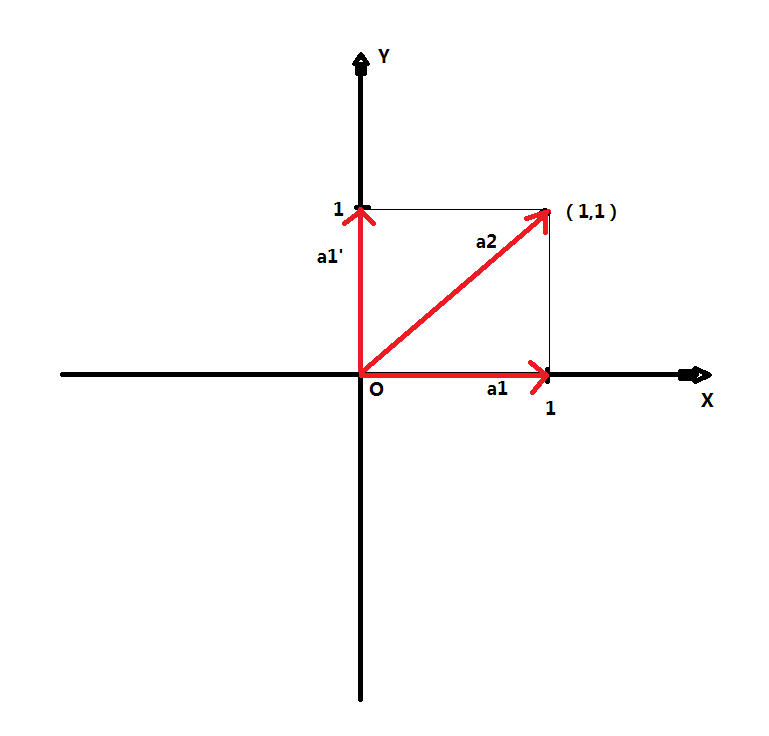我们上面说了a1,a2不垂直，那么他们就是某些分量有冗余的，从这个图就能看出来，a2,a1向量夹角明显小于90度，但是因为a1,a2线性无关，所以可以作为一组基，能表示出这个平面中的任何一个向量。
但是现在我们需要在a1,a2向量中找出正交的两个向量e1,e2作为新的一组基，即正交基（因为正交基形式简单，具有很多优良性质，所以数学家需要找到这组基）
我们先在a1的基础上进行，令e1=a1/|a1|，即我们先单位化（也叫做标准化）a1，然后这就是成为了第一个标准正交基，从刚刚的想法，应该是在a2中扣除与a1的冗余部分，那么剩下的向量部分肯定就是与a1正交的了（实际上就是我们把a2向量再正交分解一次，分解为为冗余的a1和垂直于a1的a1‘向量），从图中可以看出，a2由两部分构成，a1和a1'向量（这个向量实际上就是垂直于a1的），也就是：a2 = a1 + a1'，但是我们写为更一般的形式，
a2 = c*e1 + a1'，（注：因为我们已经令e1=a1/|a1|，即e1已经是单位化的了，所以a2在a1向量方向上的单位分量肯定得带一个系数c，刚好我举的这个例子这里c=1罢了），现在我们只要能找出这个a1’向量（因为前面没带系数，那么我们找到的a1‘向量可能不是单位化的，我们一定得正交化一次，才能成为第二个标准正交基）即可，
也就是找到了a2中蕴藏的第二个正交基 e2 = a1'/|a1'|
根据a2 = c*e1 + a1'，推出
a1' = a2 - c*e1 即为我们所求，这里因为c是未知系数，我们是求不出a1’的，因此我们得先求出系数c即可
再结合一个条件：a1'得垂直于e1，即使得a1' * e1 = 0（他两内积得为零）
现在我们带a1' = a2 - c*e1进入a1' * e1 = 0，得到：
（a2 - c*e1）*e1 =0 ，展开得到
a2*e1 = c*e1*e1，因为e1*e1 = 1（单位向量与自己内积当然是等于模长啦！就是=1的）即
c = a2*e1 = (a2,e1) （写成了向量内积的括号表示方式）
由于a2，e1都是已知的向量，所以这里我们就求出了系数c，那么结合上面的式子a1' = a2 - c*e1就可以求得第二个正交向量，
带入向量的值，a2 = （1,1），e1 = （1,0），计算得到：
a1' = a2 - (a2,e1)*e1 = a2 - e1 = （0,1）
此时再单位化得到的第二个正交基a1'向量，e2 = a1' / |a1'| = （0,1） / 1 = （0,1），就得到了我们的第二个标准正交基
哈哈，是不是发现这个（0,1）向量正好是图中那个y轴上面的与a1垂直的a1'向量，那这就对了，虽然举的这个例子比较简单，而且参数也比较特殊了一点，但是足以说明这个问题的本质了，你还可以试着自己举一些不同方向，不同长度的a1，a2向量，然后安照这个原理进行正交化，你会发现，完全正确！
现在e1，e2都找到了，那么这个标准正交化就结束了。
现在我们用最开始书本图片上那个正交化公式来计算一遍，看看是不是和我们这个结果吻合的：
β1 = a1，（我刚刚标准正交基向量用的是e1，e2的符号，书上这里用的是β1，β2，不过没关系，知道就好）
β2 = a2 - （β1，a2） / （β1，β1） * β1
带入向量的值，a2 = （1,1），β1 = a1 = （1,0），计算得到：
β2 = （1,1）- 1/1 * （1,0） = （0,1）
这个结果跟咱们刚刚那个绿色的结果比较一下，是不是发现完全一样，哈哈，这就说明咱们最上面那个紫色的那段话的思想是完全正确的，恭喜你，要是时间退回两百年前，那么发明这个算法的数学家就是你了，哈哈！
现在我们已经掌握了这个正交化的原理以及精髓，现在我们需要做更一般的推理，推广到高维向量空间（什么？空间是啥你不知道？好吧，我解释一下，空间就是集合的别名，比如数轴上的所有全体实数组成的集合，我们叫做实数集对吧，我们还可以叫做实数空间，懂了吧！！！）
现在为了表述更清晰，向量我又换了几个符号来表示，不过这个没关系，聪明的你总是能分辨白天黑夜的！！！
符号说明：这里用g1，g2，g3..gn来代表上面的线性无关向量组a1,a2,a3,...an。h1,h2...hn代表正交后得到的向量a1',a2'...an'
最终的单位正交向量还是用e1,e2,...en表示。废话不多说，咱们开始，前方高能，请认真阅读，不懂的联系我qq2488890051.
设{ g1,g2,g3,...gn }为n维空间中的一组线性无关元素系
令e1 = g1 / |g1|，作h2 = g2 - c21*e1（这儿就是扣除第一个正交基的冗余部分），求出c21，利用条件h2⊥e1，即
（h2，e1） = （g2，e1）- （c21*e1，e1） = 0
故
c21 = （g2，e1），即可求得上面的h2
令e2 = h2 / |h2|，作h3 = g3 - （c31*e1 + c32*e2）（即：我们最开始说的，得扣除和之前向量e1，e2冗余的部分，只是那时候咱们是二维而已），求c31，c32，使得，h3⊥e1，h3⊥e2，可求得：
c31 = （g3，e1），c32 = （g3，e2），即可求得h3
令e3 = h3 / |h3|，..........
以此类推。
设e（n-1）（注：这里n-1是下标，为了书写方便，我加了个括号，即第n-1个标准正交基）已得，
作 hn = gn - （cn1*e1+ cn2*e2+......c（n-1）*e（n-1））（注：即为扣除前面所有的冗余向量）
使 hn⊥e1，hn⊥e2，hn⊥e3.......hn⊥e（n-1），求得cn1，cn2，cn3.......c（n-1）等系数，即：
cn1 = （gn，e1），cn2 = （gn，e2），cn3 = （gn，e3）.....cn(n-1) = (gn，e（n-1））
即可求得hn
令 en = hn / |hn|，.......
由此而得到的{e1，e2，e3......en}即为由{g1，g2，g3......gn}标准正交化（也叫做规范正交化）而得到的标准正交系（规范正交系）。
这里讲一下，上面这个红色加粗字体，系数的来源的通熟易懂的直观理解，前面我们用的是
h2 = g2 - c21*e1，求出c21，利用条件h2⊥e1，即（h2，e1） = （g2，e1）- （c21*e1，e1） = 0
故c21 = （g2，e1），从而求出的这个系数，这个是严格的推导而来，没有任何问题。现在我们来如下思考，就更加容易结合实际意义来理解了。
若A = c1*a1 + c2*a2 + c3*a3 + ...cn*an  这里A是更一般的（即更抽象性，代表了不同事物的相同本质的东西），A可能是个向量，也可能是一个函数。这里面a1,a2,a3,......an互相垂直的一组标准正交基，即内积为零，函数也是有基函数，有内积的，所以这里说的东西适用于向量和函数，c1，c2...cn为这些基的系数。现在假如我们想求A在某一个基（分量）的系数，那么怎么求呢，实际上就是用 cn = （an，A），即用an去与A作内积，由于这些基两两正交，所以必然只有an * an这一项不为零，那么
（an，A） = an*cn*an = cn*1 = cn 这个系数了嘛（an*an = 1的，因为是标准正交基，模长为1），上面红色加粗字体部分我们求向量的系数是如此，实际上求函数的标准正交基的系数也是如此，下面我举一个函数的例子：
我们知道傅立叶变换中，满足狄利克雷条件的f（x）都可以展开成傅立叶级数，
即1，cosx，sinx，cos2x，sin2x......cosnx，sinnx，...（因为他们是一组完备的正交基，什么是完备？？？哈哈，就是你再也找不到另外一个基函数可以与他们两两相交的，他们就已经是整个宇宙中能找到的最完整的一组正交基了，但是他们为啥不是标准正交基？我们来积分一下，因为∫cosxdx从-pi到pi积分 = pi，而不是1。不过这个没关系，我们把它除以一个pi就可以将他们单位化了，即1/π，1/π*cosx，1/π*sinx，1/π*cos2x，1/π*sin2x......1/π*cosnx，1/π*sinnx，...他们现在就是一组标准正交基函数了）
我们现在把f（x）展开成三角函数的标准正交基的表示形式，这里用an表示cosnx的系数，bn表示sinnx的系数，即：
f（x） = a0*1/π*cos0x + b0*1/π*sin0x(注：这一项=0) + a1*1/π*cosx + b1*1/π*sinx + a2 * 1/π*cos2x + b2*1/π*sin2x + ...... + an*1/π*cosnx + bn*1/π*sinnx
即：
f（x） = a0*1/π + a1*1/π*cosx + b1*1/π*sinx + a2 * 1/π*cos2x + b2*1/π*sin2x + ...... + an*1/π*cosnx + bn*1/π*sinnx）
根据上面粉红色的字体部分说的，求某个正交基上面的系数的方法就是用这个正交基去内积f（x）即可，即：
a1 = (1/π*cosx，f(x)）= ∫f(x)*1/π*cosxdx，                b1 = (1/π*sinx，f(x) ）= ∫f(x)*1/π*sinxdx
a2 = (1/π*cos2x，f(x)）= ∫f(x)*1/π*cos2xdx，            b2 = (1/π*sin2x，f(x)）= ∫f(x)*1/π*sin2xdx
......
an = (1/π*cosnx，f(x)）= ∫f(x)*1/π*cosnxdx，            bn = (1/π*sinnx，f(x)）= ∫f(x)*1/π*sinnxdx
即：
an = 1/π*∫f(x) * cosnxdx       (n =0,1,2,3....)，特别的，当n=0时，a0 = 1/π∫f(x)dx
bn = 1/π*∫f(x) * sinnxdx        (n =1,2,3....)
哈哈，这就是傅立叶变换后，系数的求解方法，大佬，你记住了吗！！！

展开全文线性代数
• 标准的定义引自Wiki We define the projection operator by proju(v)=⟨v,u⟩⟨u,u⟩uproj_u(v) = \frac{ \langle v,u\rangle }{\langle u, u \rangle}u where ⟨v,u⟩\langle v, u \rangle denotes the ...
先看一个例子：  设三个向量分别为：

α1α2α3=(1,−1,0)T=(1,0,−1)T=(1,1,1)T

\begin{align}\alpha_1 &=(1,-1,0)^T\\
\alpha_2 & = (1,0,-1)^T\\
\alpha_3 & = (1,1,1)^T
\end{align}
那么对

α1,α2
$\alpha_1,\alpha_2$ 正交化：

β1β2=α1=(1,−1,0)T=α2−(α2,β1)(β1,β1)β1=(1,0,−1)T−1×1+0×(−1)+(−1)×01×1+(−1)×(−1)+0×0(1,−1,0)T=(1,0,−1)T−12(1,−1,0)T=12(1,1,−2)T

\begin{align}
\beta_1 & = \alpha_1=(1,-1,0)^T\\
\beta_2 & = \alpha_2-\frac{(\alpha_2, \beta_1)}{(\beta_1, \beta_1)}\beta_1\\
& = (1,0,-1)^T-\frac{1\times1+0\times(-1)+(-1)\times0}{1\times1+(-1)\times(-1)+0\times0}(1,-1,0)^T\\
& = (1,0,-1)^T - \frac{1}{2}(1,-1,0)^T\\
& = \frac{1}{2}(1,1,-2)^T
\end{align}
再对

β1,β2,β3
$\beta_1,\beta_2,\beta_3$ 单位化：

γ1γ2γ3=12‾‾√(1,−1,0)T=16‾‾√(1,1,−2)T=13‾‾√(1,1,1)T

\begin{align}
\gamma_1 & = \frac{1}{\sqrt{2}}(1,-1,0)^T\\
\gamma_2 & = \frac{1}{\sqrt{6}}(1,1,-2)^T\\
\gamma_3 & = \frac{1}{\sqrt{3}}(1,1,1)^T
\end{align}

以下施密特正交化的标准定义引自Wiki

We define the projection operator by

proju(v)=⟨v,u⟩⟨u,u⟩u

proj_u(v) = \frac{ \langle v,u\rangle }{\langle u, u \rangle}u
where

⟨v,u⟩
$\langle v, u \rangle$ denotes the inner product of the vectors

v
$\mathbf v$ and

u
$\mathbf u$. This operator projects the vector

v
$\mathbf v$ orthogonally onto the line spanned by vector

u
$\mathbf u$. If

u=0
$\mathbf u=0$, we define

proj0(v):=0
$proj_0(v):=0$. i.e., the procjection map

proj0
$proj_0$ is the zero map, sending every vector to the zero vector.
The Gram-Schmidt process then works as follows:

u1u2u3u4uk=v1=v2−proju1(v2)=v3−proju1(v3)−proju2(v3),=v4−proju1(v4)−proju2(v4)−proju3(v4),⋮=vk−∑j=1k−1projuj(vk),e1=u1∥u1∥e2=u2∥u2∥e3=u3∥u3∥e3=u4∥u4∥ek=uk∥uk∥

\begin{align}
u_1 & =v_1 & e_1=\frac{u_1}{\Vert u_1 \Vert}\\
u_2 & =v_2-proj_{u_1}(v_2) & e_2=\frac{u_2}{\Vert u_2 \Vert}\\
u_3 & =v_3-proj_{u_1}(v_3)-proj_{u_2}(v_3), & e_3=\frac{u_3}{\Vert u_3 \Vert}\\
u_4 & =v_4-proj_{u_1}(v_4)-proj_{u_2}(v_4)-proj_{u_3}(v_4), & e_3=\frac{u_4}{\Vert u_4 \Vert}\\
&\vdots \\
u_k & =v_k-\sum_{j=1}^{k-1}proj_{u_j}(v_k), & e_k=\frac{u_k}{\Vert u_k \Vert}
\end{align}

The sequence

u1,...,uk
$u_1, ..., u_k$ is the required system of orthogonal vectors, and the normalized vectors

e1,...,ek
$e_1, ..., e_k$ from an orthonormal set. The calculation of the sequence

u1,...,uk
$u_1, ..., u_k$ is known as
Gram-Schmidt rothogonalization, while the calculation of the sequence

e1,...,ek
$e_1, ..., e_k$ is known as
Gram-Schmidt orthonormalization as the vectors are normalized.

部分符号和国内教材上有差异。
展开全文数学 线性代数
• 正交向量正交化线性相关正交向量正交化 线性相关 定义 定义1：在向量空间 VVV 的一组向量 AAA ：α1,α2,⋯αm\alpha_{1},\alpha_{2},\cdots \alpha_{m}α1​,α2​,⋯αm​，如果存在不全为零的数 k1,k2,⋯...


正交向量与正交化
线性相关正交向量正交基正交化

线性相关

定义
定义1：在向量空间

V

V

的一组向量

A

A

：

α

1

,

α

2

,

⋯

α

m

\alpha_{1},\alpha_{2},\cdots \alpha_{m}

，如果存在不全为零的数

k

1

,

k

2

,

⋯

k

m

k_{1},k_{2},\cdots k_{m}

，使

k

1

α

1

+

k

2

α

2

+

⋯

+

k

m

α

m

=

0

k_{1}\alpha_{1}+k_{2}\alpha_{2}+\cdots +k_{m}\alpha_{m}=0

则称向量组

A

A

是线性相关的，否则，称其为线性无关的。定义2：当且仅当

α

1

,

α

2

,

⋯

α

m

\alpha_{1},\alpha_{2},\cdots \alpha_{m}

全为零时，使得使

k

1

α

1

+

k

2

α

2

+

⋯

+

k

m

α

m

=

0

k_{1}\alpha_{1}+k_{2}\alpha_{2}+\cdots +k_{m}\alpha_{m}=0

成立，称向量组线性无关。

定理：
两个向量

a

a

、

b

b

共线的充要条件是

a

a

、

b

b

线性相关；三个向量

a

a

、

b

b

、

c

c

共面的充要条件是

a

a

、

b

b

、

c

c

线性相关；向量

a

1

,

a

2

,

⋅

⋅

⋅

,

a

n

(

n

⩾

2

)

a_1,a_2, ···,a_n (n\geqslant 2)

线性相关的充要条件是这

n

n

个向量中的一个为其余

(

n

−

1

)

(n-1)

个向量的线性组合，即有

a

n

a_n

，且存在数域K中一组数

k

1

,

k

2

,

⋯

k

m

k_{1},k_{2},\cdots k_{m}

，满足

a

n

=

k

1

a

1

+

k

2

a

2

+

⋯

+

k

n

−

1

a

n

−

1

a_n = k_1a_1+k_2a_2 + \cdots +k_{n-1}a_{n-1}

注意：
对于任一向量组而言,，不是线性无关的就是线性相关的；包含零向量的任何向量组是线性相关的；含有相互平行的向量的向量组必线性相关；向量组是线性相关的，那么增加向量的个数，不改变向量的相关性。【局部相关，整体相关】向量组是线性无关的，那么减少向量的个数，不改变向量的无关性。【整体无关，局部无关】
正交向量
通常，两个向量垂直的充分必要条件是它们夹角的余弦为零，亦即它们的数量积为零。在一般的欧式空间中，仍以内积定义两向量夹角的余弦。

定义：如果对于欧式空间中的两个向量

x

x

与

y

y

有

(

x

,

y

)

=

0

(x,y)=0

，则称

x

x

与

y

y

正交 或 垂直 ，记为

x

⊥

y

x\perp y

。

结论：
当

x

x

与

y

y

正交时，

y

y

与

x

x

也正交；零向量与任意向量均正交；如果

x

⊥

y

x\perp y

，且

x

x

与

y

y

线性相关，则此二向量中至少有一个是零向量。

定义：如果欧式空间中一组非零向量两两正交，则称为正交向量组。
定理：设

x

1

,

x

2

,

⋯

x

m

x_{1},x_{2},\cdots x_{m}

是正交向量组，则它们必线性无关。

上述定理可以表明，在

n

n

维欧式空间中，两两正交的非零向量不能超过

n

n

个。例如，在平面上找不到三个两两正交的非零向量；在通常的三维空间

R

3

R^3

中，找不到四个两两正交的非零向量。
正交基

定义：在欧式空间

V

n

V^n

中，由

n

n

个非零向量组成的正交向量组称为

V

n

V^n

的 正交基 ；由单位向量组成的正交基称为 标准正交基 或 法正交基 ；其基向量称为 单位坐标向量 。

一个基为标准正交基的 充要条件 是它的度量矩阵为单位矩阵。
证明：设

x

1

,

x

2

,

⋯

x

n

x_{1},x_{2},\cdots x_{n}

为标准正交基，则由定义有

(

x

i

,

x

j

)

=

x

i

T

x

j

=

{

1

if

i

=

j

0

if

i

≠

j

\left ( x_{i},x_{j} \right )=x_{i}^{T}x_{j}=\begin{cases} 1 & \text{ if } i=j \\ 0 & \text{ if } i\neq j \end{cases}

可见 ，它的度量矩阵是单位矩阵。反之，如果以单位矩阵为度量矩阵，则由矩阵相等可得

(

x

i

,

x

j

)

=

x

i

T

x

j

\left ( x_{i},x_{j} \right )=x_{i}^{T}x_{j}

，即

x

1

,

x

2

,

⋯

x

n

x_{1},x_{2},\cdots x_{n}

为标准正交基。
正交化

定理：对于欧式空间

V

n

V^n

的任一基

x

1

,

x

2

,

⋯

x

n

x_{1},x_{2},\cdots x_{n}

，都可以找到一个标准正交基

y

1

,

y

2

,

⋯

y

n

y_{1},y_{2},\cdots y_{n}

。换言之，任一非零欧式空间都有正交基和标准正交基。

这个正交基我们可以通过Gram-Schmidt正交化获得。
Gram-Schmidt正交化方法是将 线性无关 的向量转化为 标准正交化向量 的方法。注意这里的前提，Gram-Schmidt正交化方法是对线性无关的向量操作。

假设欧式空间

V

n

V^n

中的任一基为

x

1

,

x

2

,

⋯

x

n

x_{1},x_{2},\cdots x_{n}

，我们通过Gram-Schmidt正交化方法得到该基的正交基和标准正交基。
取

β

1

=

x

1

\beta_{1}=x_1

，作所求正交基中的第一个向量。令

β

2

=

x

2

+

k

β

1

\beta_{2}=x_2+k\beta_{1}

，由于我们要构造的正交基两两向量相互正交，有

(

β

1

,

β

2

)

=

0

(\beta_{1},\beta_{2})=0

，通过这个条件来计算待定常数

k

k

。由

(

β

1

,

x

2

+

k

β

1

)

=

(

β

1

,

x

2

)

+

k

(

β

1

,

β

1

)

=

0

(\beta_{1},x_2+k\beta_{1})=(\beta_{1},x_2)+k(\beta_{1},\beta_{1})=0

得

k

=

−

(

x

2

,

β

1

)

(

β

1

,

β

1

)

k=-\frac{(x_2,\beta _{1})}{(\beta _{1},\beta _{1})}

代入

β

2

=

x

2

+

k

β

1

\beta_{2}=x_2+k\beta_{1}

，这样就得到两个相互正交的向量

β

1

\beta_{1}

，

β

2

\beta_{2}

，且

β

2

≠

0

\beta_{2} \neq 0

。令

β

3

=

x

3

+

k

2

β

2

+

k

1

β

1

\beta_{3}=x_3+k_2\beta_{2}+k_1\beta_{1}

，再由正交条件

(

β

1

,

β

3

)

=

0

(\beta_{1},\beta_{3})=0

及

(

β

2

,

β

3

)

=

0

(\beta_{2},\beta_{3})=0

来决定出常数

k

1

k_1

和

k

2

k_2

为

k

2

=

−

(

x

3

,

β

2

)

(

β

2

,

β

2

)

,

k

1

=

−

(

x

3

,

β

1

)

(

β

1

,

β

1

)

k_2=-\frac{(x_3,\beta _{2})}{(\beta _{2},\beta _{2})},k_1=-\frac{(x_3,\beta _{1})}{(\beta _{1},\beta _{1})}

以此类推，继续进行下去，直到最后一个向量。

上述获得的向量组

(

β

1

,

β

2

,

⋯

,

β

n

)

(\beta _{1},\beta _{2},\cdots ,\beta _{n})

即为欧式空间

V

n

V^n

中的一个 正交基 。再将各向量单位化，即除以各自的模，得到单位正交向量组

y

1

,

y

2

,

⋯

y

n

y_{1},y_{2},\cdots y_{n}

，也即 标准正交基。

y

i

=

β

i

∣

β

i

∣

,

i

=

1

,

2

,

.

.

.

,

n

y_{i}=\frac{\beta_i}{\left | \beta _{i} \right |},i=1,2,...,n

由基

x

1

,

x

2

,

⋯

x

n

x_{1},x_{2},\cdots x_{n}

求标准正交基

y

1

,

y

2

,

⋯

y

n

y_{1},y_{2},\cdots y_{n}

的过程也称把基

x

1

,

x

2

,

⋯

x

n

x_{1},x_{2},\cdots x_{n}

正交单位化 或 正交规范化 。
为简单起见，以包含四个向量的向量组为例，介绍Schmidt正交化的具体过程。假设向量组为

x

1

=

(

1

,

1

,

0

,

0

)

x_{1}=\left ( 1,1,0,0 \right )

，

x

2

=

(

1

,

0

,

1

,

0

)

x_{2}=\left ( 1,0,1,0 \right )

，

x

3

=

(

−

1

,

0

,

0

,

1

)

x_{3}=\left ( -1,0,0,1 \right )

，

x

4

=

(

1

,

−

1

,

−

1

,

1

)

x_{4}=\left ( 1,-1,-1,1 \right )

，求解其标准正交基。
解：首先先把它们正交化。 取

β

1

=

x

1

=

(

1

,

1

,

0

,

0

)

\beta_{1}=x_1=\left ( 1,1,0,0 \right )

令

β

2

=

x

2

+

k

β

1

\beta_{2}=x_2+k\beta_{1}

，常数

k

k

为

k

=

−

(

x

2

,

β

1

)

(

β

1

,

β

1

)

=

−

1

2

k=-\frac{(x_2,\beta _{1})}{(\beta _{1},\beta _{1})}=-\frac{1}{2}

，则

β

2

=

x

2

+

k

β

1

=

(

1

,

0

,

1

,

0

)

−

1

2

(

1

,

1

,

0

,

0

)

=

(

1

2

,

−

1

2

,

1

,

0

)

\begin{aligned} \beta_{2}&=x_2+k\beta_{1} \\ &=\left ( 1,0,1,0 \right )-\frac{1}{2}\left ( 1,1,0,0 \right )\\ &=(\frac{1}{2},-\frac{1}{2},1,0) \end{aligned}

令

β

3

=

x

3

+

k

2

β

2

+

k

1

β

1

\beta_{3}=x_3+k_2\beta_{2}+k_1\beta_{1}

，常数

k

1

k_1

与

k

2

k_2

为

k

1

=

−

(

x

3

,

β

1

)

(

β

1

,

β

1

)

=

1

2

,

k

2

=

−

(

x

3

,

β

2

)

(

β

2

,

β

2

)

=

−

−

1

/

2

3

/

2

=

1

3

k_1=-\frac{(x_3,\beta _{1})}{(\beta _{1},\beta _{1})}=\frac{1}{2},k_2=-\frac{(x_3,\beta _{2})}{(\beta _{2},\beta _{2})}=-\frac{-1/2}{3/2}=\frac{1}{3}

， 则

β

3

=

x

3

+

k

2

β

2

+

k

1

β

1

=

(

−

1

,

0

,

0

,

1

)

+

1

3

(

1

2

,

−

1

2

,

1

,

0

)

+

1

2

(

1

,

1

,

0

,

0

)

=

(

−

1

3

,

1

3

,

1

3

,

1

)

\begin{aligned} \beta_{3} &= x_3+k_2\beta_{2}+k_1\beta_{1}\\ &= \left ( -1,0,0,1 \right )+\frac{1}{3}(\frac{1}{2},-\frac{1}{2},1,0)+\frac{1}{2}\left ( 1,1,0,0 \right )\\ &= (-\frac{1}{3},\frac{1}{3},\frac{1}{3},1) \end{aligned}

令

β

4

=

x

4

+

k

3

β

3

+

k

2

β

2

+

k

1

β

1

\beta_{4}=x_4+k_3\beta_{3}+k_2\beta_{2}+k_1\beta_{1}

，常数

k

1

k_1

与

k

2

k_2

为

k

1

=

−

(

x

4

,

β

1

)

(

β

1

,

β

1

)

=

0

,

k

2

=

−

(

x

4

,

β

2

)

(

β

2

,

β

2

)

=

0

，

k

3

=

−

(

x

4

,

β

3

)

(

β

3

,

β

3

)

=

0

k_1=-\frac{(x_4,\beta _{1})}{(\beta _{1},\beta _{1})}=0,k_2=-\frac{(x_4,\beta _{2})}{(\beta _{2},\beta _{2})}=0，k_3=-\frac{(x_4,\beta _{3})}{(\beta _{3},\beta _{3})}=0

， 则

β

4

=

x

4

+

k

3

β

3

+

k

2

β

2

+

k

1

β

1

=

(

1

,

−

1

,

−

1

,

1

)

\beta_{4}=x_4+k_3\beta_{3}+k_2\beta_{2}+k_1\beta_{1}= ( 1,-1,-1,1 )

再单位化，便有

y

1

=

β

1

∣

β

1

∣

=

1

2

β

1

=

(

1

2

,

1

2

,

0

,

0

)

y_{1}=\frac{\beta_1}{\left | \beta _{1} \right |}=\frac{1}{\sqrt{2}}\beta _{1}=(\frac{1}{\sqrt{2}},\frac{1}{\sqrt{2}},0,0)

y

2

=

β

2

∣

β

2

∣

=

2

6

β

2

=

(

1

6

,

−

1

6

,

2

6

,

0

)

y_{2}=\frac{\beta_2}{\left | \beta _{2} \right |}=\frac{2}{\sqrt{6}}\beta _{2}=(\frac{1}{\sqrt{6}},-\frac{1}{\sqrt{6}},\frac{2}{\sqrt{6}},0)

y

3

=

β

3

∣

β

3

∣

=

3

2

β

3

=

(

−

1

12

,

1

12

,

1

12

,

3

12

)

y_{3}=\frac{\beta_3}{\left | \beta _{3} \right |}=\frac{\sqrt{3}}{2}\beta _{3}=(-\frac{1}{\sqrt{12}},\frac{1}{\sqrt{12}},\frac{1}{\sqrt{12}},\frac{3}{\sqrt{12}})

y

4

=

β

4

∣

β

4

∣

=

1

2

β

4

=

(

1

2

,

−

1

2

,

−

1

2

,

1

2

)

y_{4}=\frac{\beta_4}{\left | \beta _{4} \right |}=\frac{1}{2}\beta _{4}=(\frac{1}{2},-\frac{1}{2},-\frac{1}{2},\frac{1}{2})


展开全文线性代数 矩阵
• 正交定义 正交例题 正交向量组 标准正交基 Schmidt正交化 例题
正交定义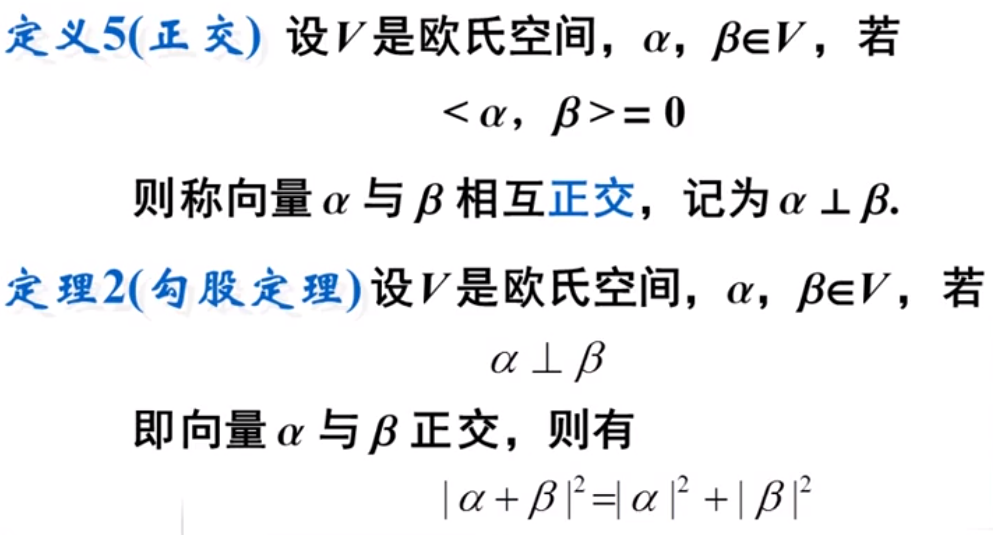正交例题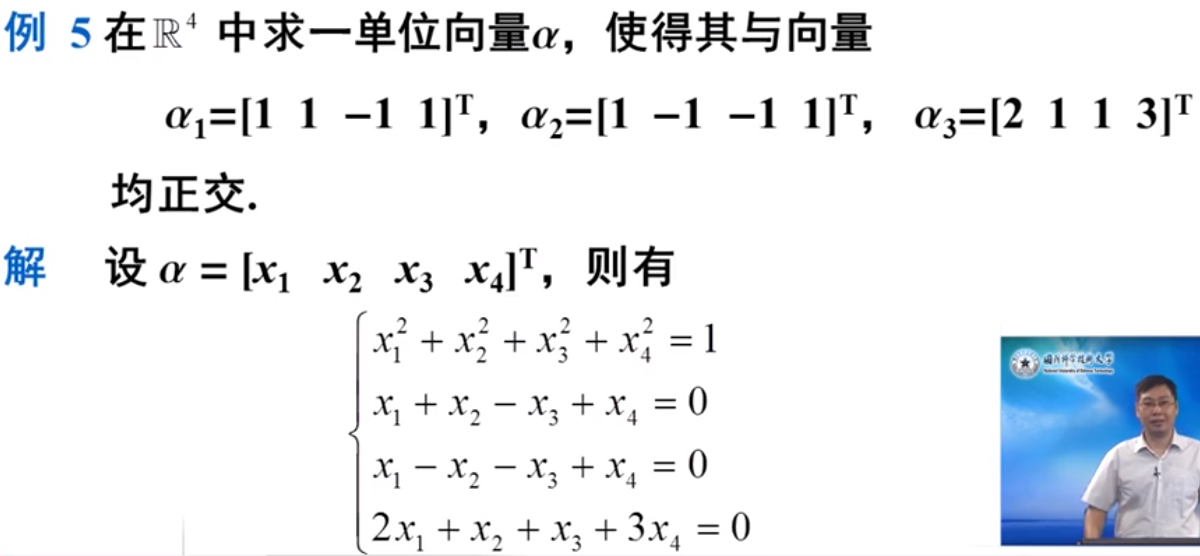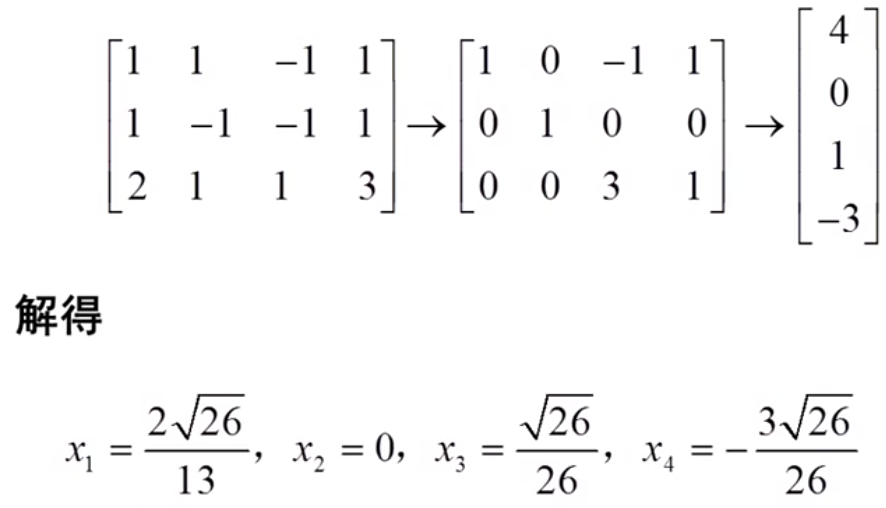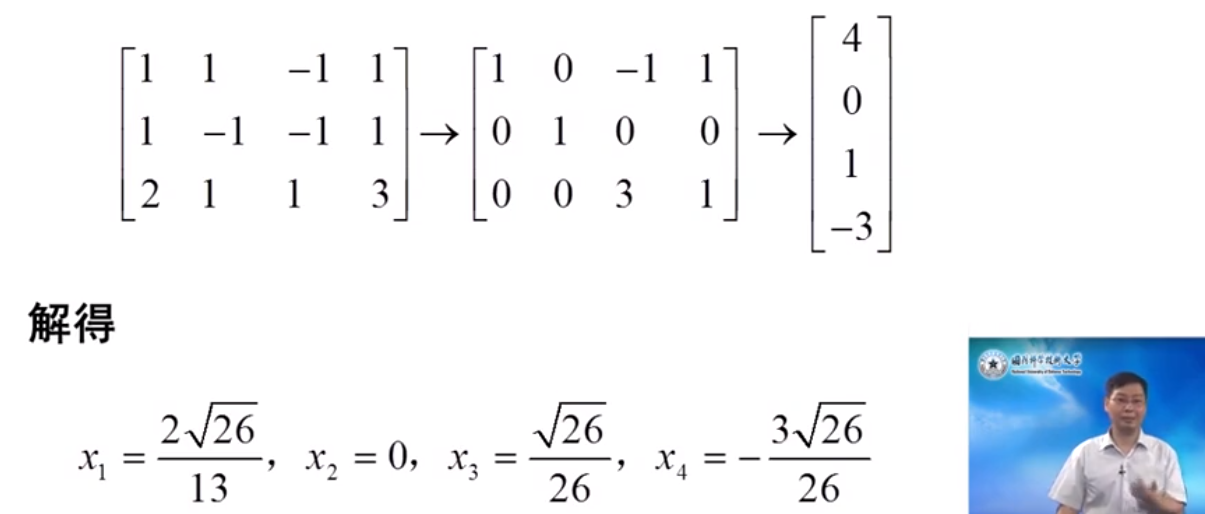正交向量组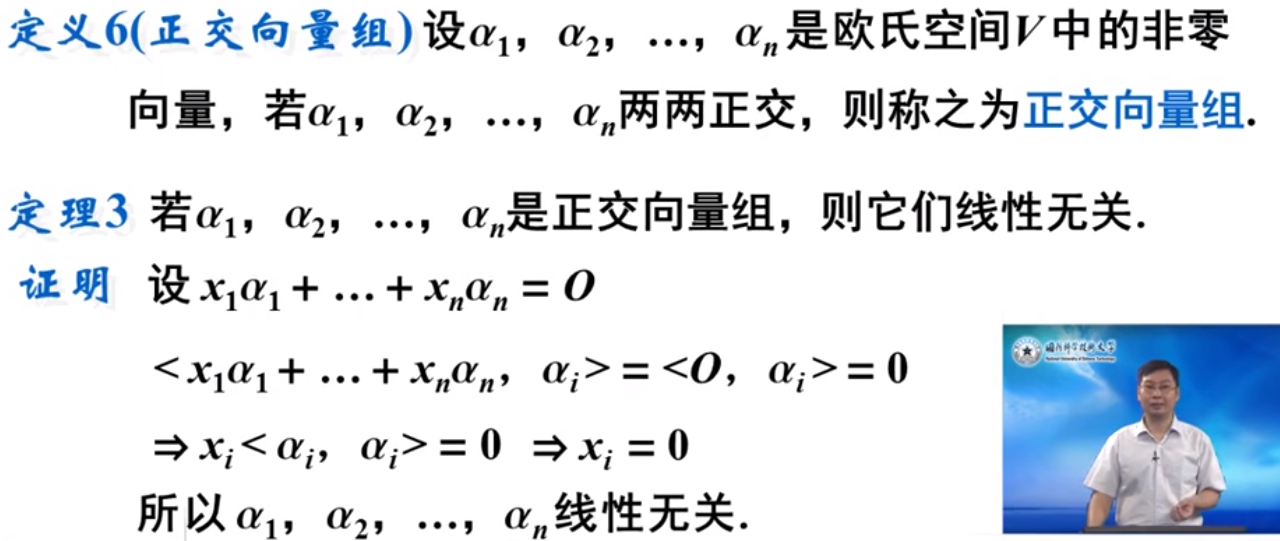标准正交基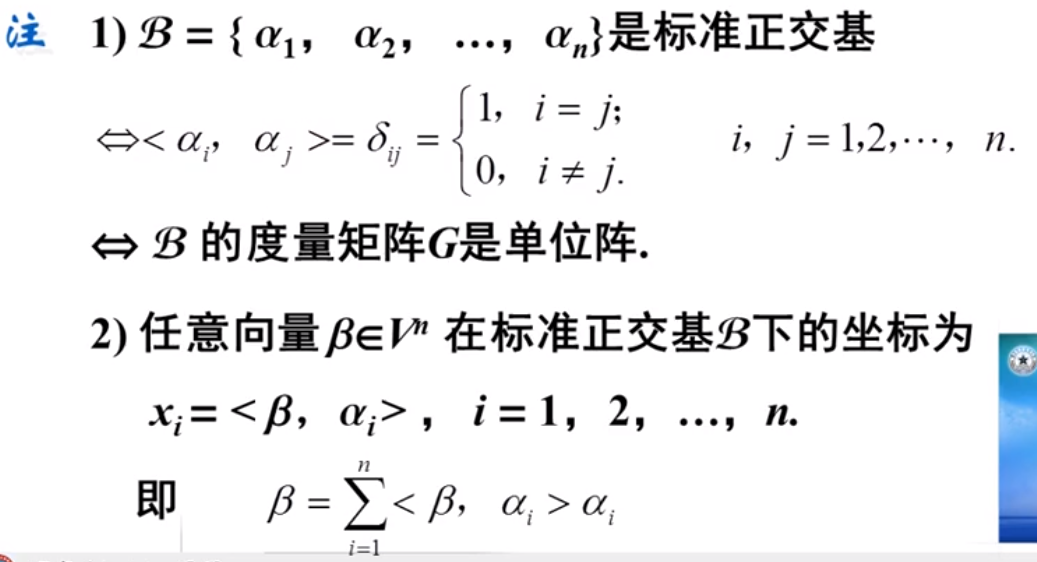Schmidt正交化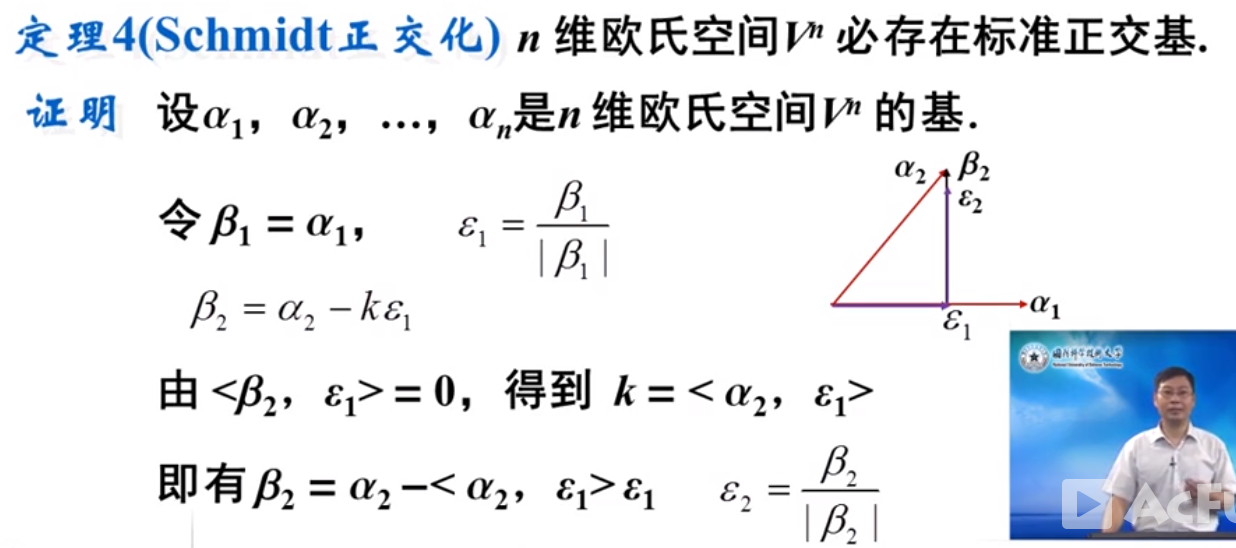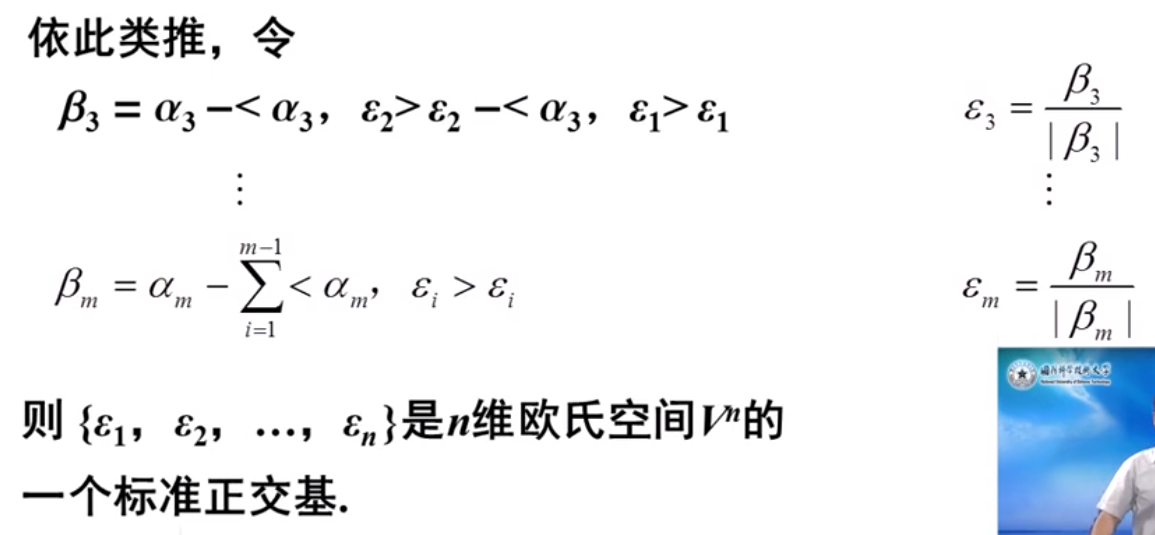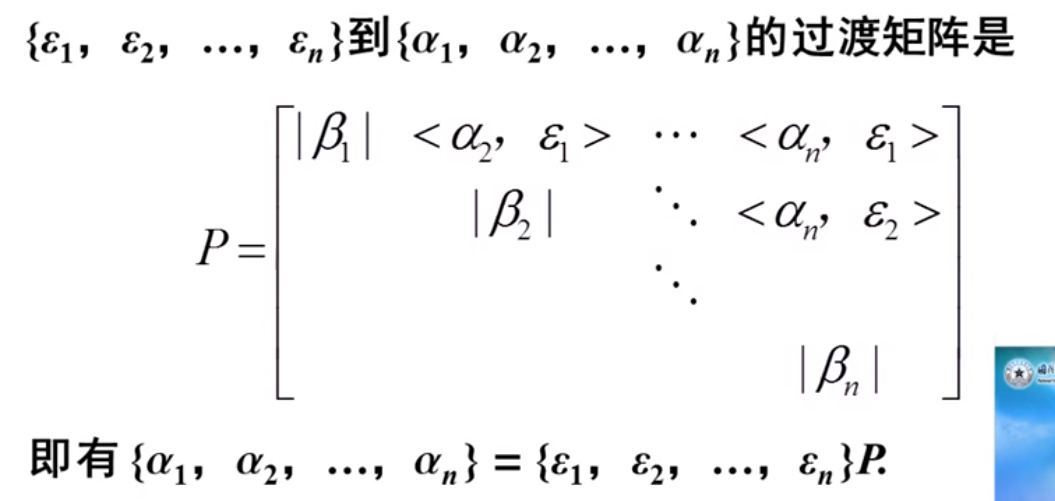例题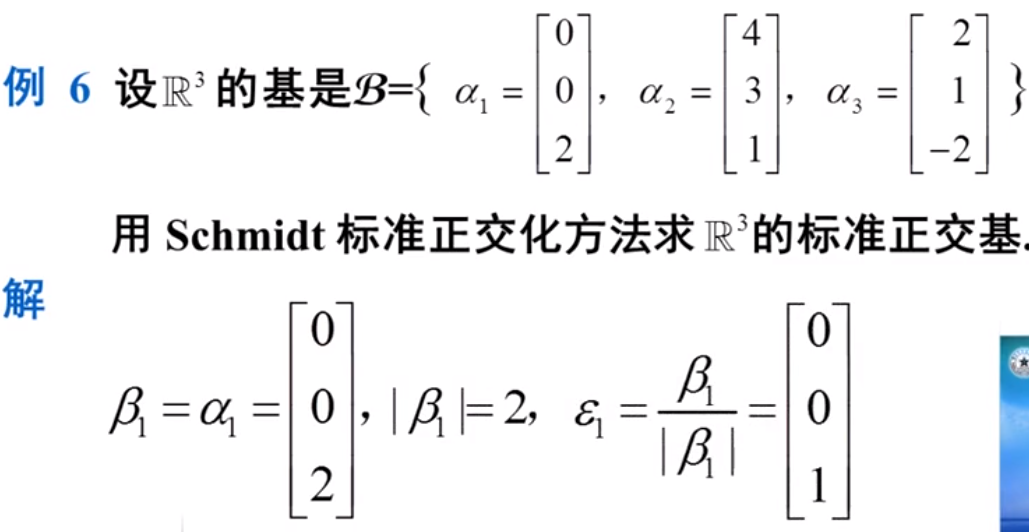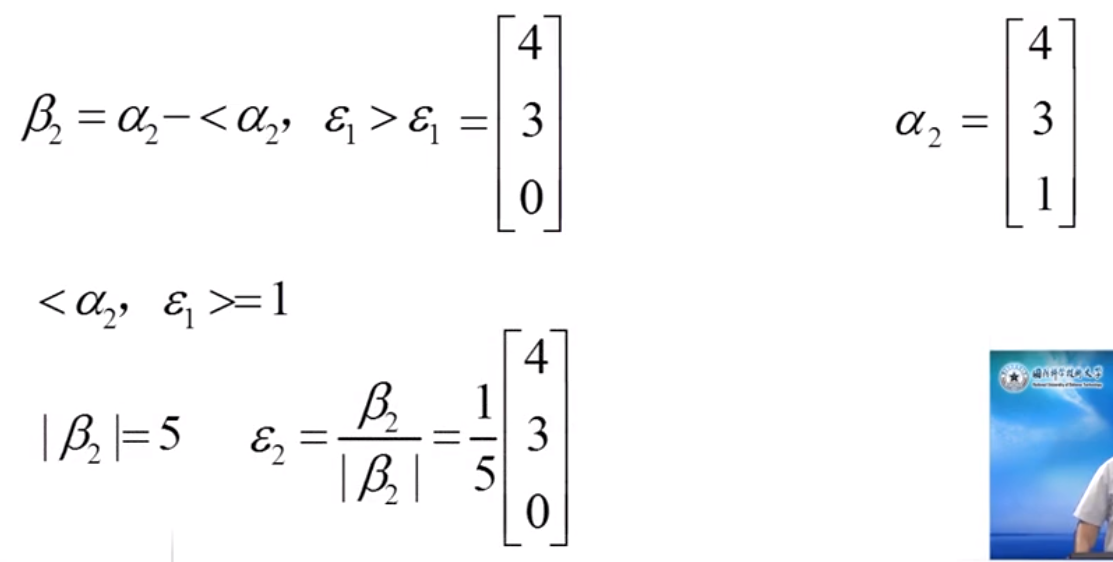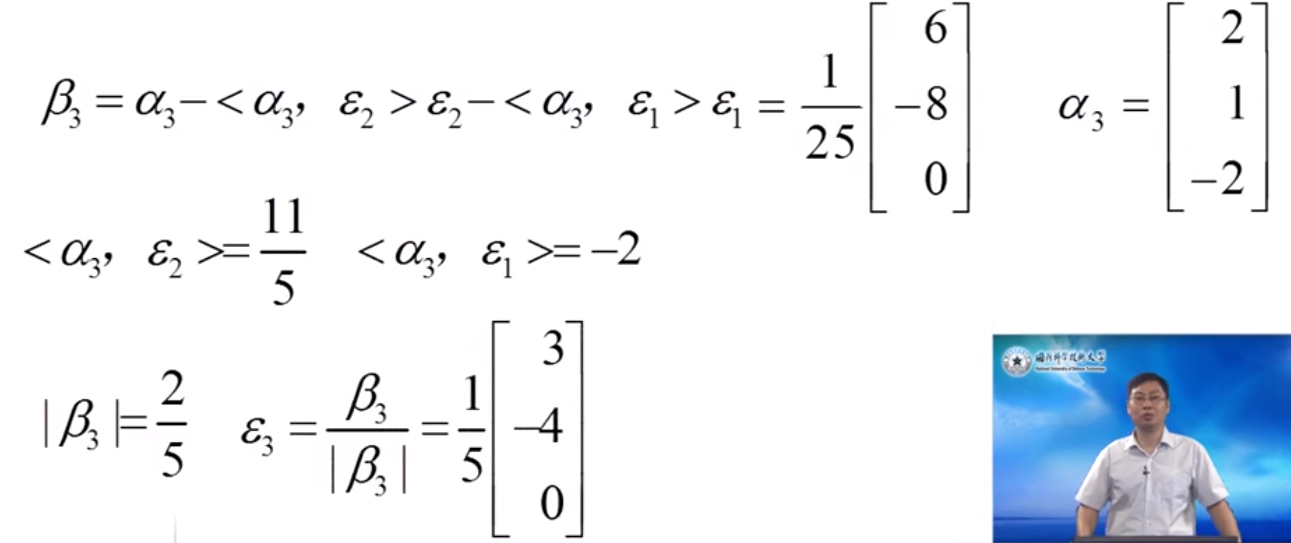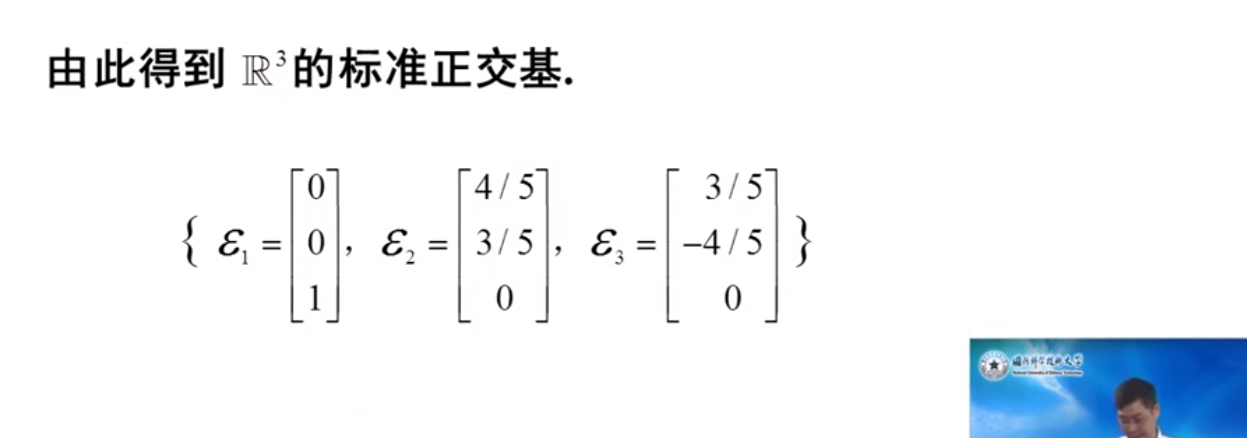展开全文矩阵论
• 本节为线性代数复习笔记的第二部分，矩阵的概念与计算（1），主要包括：向量组的秩，向量内积、正交、模，施密特标准正交化（正交规范化），向量空间以及坐标变换公式。 1. 向量组的秩   向量组α1⃗,α2⃗,...,...线性代数 数学
• 由一般向量构成的一基往往不是标准正交的，所以需要进行标准正交化！  线性代数“基“的概念在很多领域都有应用，包括机器人学，图像处理，数据降维等，具体可以参考相关线性代数教材。  本文采用C语言实现了基...C语言 数组 线性代数
• 文章目录1、向量空间1.1 向量空间的概念1.2 向量的内积1.3 向量的范数1.4 标准正交向量组1.4 Gram-Schmidt正交化1.5 向量的正交表示2、信号空间2.1 信号的能量2.2 信号的内积2.3 信号的范数2.4 信号的相关系数2.5 ...
• sympy的符号计算功能很强大，学习矩阵分析，重温了线性代数中施密特正交化的方法，正好可以用sympy解决一些计算问题。施密特正交化，也称 Gram-Schmidt 正交化过程 (Gram–Schmidt Orthogonalization Procedure). 该...sympy
• 2 向量正交 3 函数的正交 4 函数正交的意义 1 向量内积和外积 1.1 向量内积(点乘) 假设 a = [a1,a2,...,an], b = [b1,b2,...,bn]； 则a与b的内积为 a·b = a1·b1+a2·b2+...+a3·b3 = |a||b|cosθ； ...
• 线代同学的疑问: 这句话怎么去理解？我作的答疑: 由施密特正交化的过程知，施密特正交化在实对称矩阵对角化中使用，在相同特征值...从欧氏空间任意线性无关的向量组α1，α2，……，αm出发，求得正交向量组β1，β2...
• α2，……，αm出发，求得正交向量组β1，β2，……，βm，使由α1，α2，……，αm与向量组β1，β2，……，βm等价，再将正交向量组中每个向量经过单位化，就得到一个标准正交向量组，这种方法称为施密特正交化。...
• 同样的，对于一个线性变换，只要你选定一基，那么就可以找到一个矩阵来描述这个线性变换。换一基，就得到一个不同的矩阵。所有这些矩阵都是这同一个线性变换的描述，但又都不是线性变换本身。 但是这样的话，...
• ## 正交向量正交矩阵

千次阅读 2017-11-12 22:36:10
• 本节介绍正交的概念，以及将基变为正交基的格拉姆-施密特(Gram-Schmidt)方法 0 回顾正交基 1 正交 如果向量x,y满足: 则称x与y正交(orthogonal)...则称这基是标准正交的(orthonormal) 3 格拉姆-施密特方法 具有欧正交基
• α2，……，αm出发，求得正交向量组β1，β2，……，βm，使由α1，α2，……，αm与向量组β1，β2，……，βm等价，再将正交向量组中每个向量经过单位化，就得到一个标准正交向量组，这种方法称为施密特正交化。...
• %% 施密特正交化方法 format rat a1=[1 1 0 0]; a2=[1 0 1 0]; a3=[-1 0 0 1]; a4=[1 -1 -1 1]; a1 = sym( a1,'f');%定义浮点类型 b1=a1 e1=simplify(b1/norm(b1))%单位化 b2=a2-dot(a2,e1)*e...Schmidt
• 向量的内积 α\alphaα 和 β\betaβ 的内积 (α,β)(\alpha, \beta)(α,β) 为对应元素相乘再相加。内积是一个数。 向量的长度(范数、模) ∣∣α∣∣=(α,α)||\alpha||=\sqrt{(\alpha,\alpha)} ∣∣α∣∣=(α,α)...线性代数
•矩阵
• 格莱姆-施密特（Gram-Schmidt）正交化常用于求解向量空间的标准正交基，同时也是一种天然的求解矩阵的QR分解的方法，即将一个矩阵A分解为一个正交矩阵Q和一个上三角矩阵R的乘积，即A=QR。这里我们假设A是一个方阵，...QR分解
• 施密特正交化matlab程序，将矩阵的列向量进行施密特规范正交化
• 本节主要知识点 1.向量空间的定义：对加法和乘法运算封闭的非空的n维向量的集合 2.向量空间的基：向量空间中一线性无关的向量，并且其他向量可以用这组向量...5.基的正交规范向量空间的一基，两两正交而...
• 设有向量α1,α2...αn，则正交规范方法为 ... 然后，再将每个向量单位、 即 最后得到的一系列γ组成的向量组正交且均为单位向量
• 原创：量化小白H量化小白上分记本系列的第一篇因子加权方法中提到，对于因子间有相关性的...本文给出另一种更为常用的解决因子间相关性的方法：因子正交化。01背景因子多重共线性如上一篇所述，传统的多因子模型一般...
• ## 标准正交基

千次阅读 2020-01-10 12:01:06
首先，介绍内积运算，然后通过内积定义正交关系； 其次，解释了什么是基以及什么是标准正交基； 接着，阐明了标准正交基的一些便利性； 最后，给出如何由一组基得到一组标准正交基。线性代数
• 在游戏编程中我们经常会用到...α2，……，αm出发，求得正交向量组β1，β2，……，βm，使由α1，α2，……，αm与向量组β1，β2，……，βm等价，再将正交向量组中每个向量经过单位，就得到一个标准正交向量
• 1.由单个非零向量所成的向量组也是正交向量组。 2.正交向量组是线性无关的。 3.在n维欧氏空间中，两两正交的非零向量不能超过n个。 定义6 在n维欧氏空间中，由n个向量组成的正交向量组称为正交基；由单位向量...
• 标准正交矩阵 ...　向量的转置乘以自身等于1，意味着这个向量是单位向量，所以我们称这堆向量q1,q2……qn是标准正交向量标准正交矩阵 　现在把这些标准正交向量放入矩阵中： 　QTQ最终得...QR分解
• 今天我们学习一下正交向量（orthogonal vector）和正交矩阵（orthogonal matrix）。...注意准确说这组向量应该是标准正交向量（orthonormal vector），因为每个q向量长度都为1，即经过归一的（normalizatio正交矩阵...